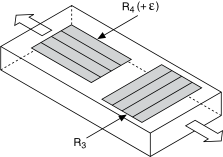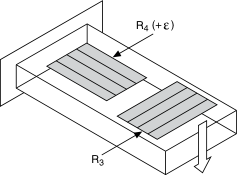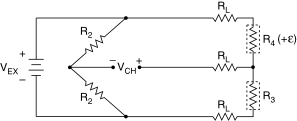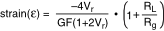# Quarter-Bridge Type II

## NI-DAQmx 18.6 Help

Edition Date: January 2019
Part Number: 370466AH-01
View Product InfoNI-DAQmx 18.6 HelpNI-DAQmx 19.0 HelpNI-DAQmx 19.1 HelpNI-DAQmx 19.5 Help

The following figure shows how to position a strain gage resistor in an axial configuration for the quarter-bridge type II.The following figure shows how to position a strain gage resistor in a bending configuration for the quarter-bridge type II.Quarter-bridge type II strain gage configurations have the following characteristics:
• One active strain gage element and one passive, temperature-sensing quarter-bridge element, known as a dummy resistor. The active element is mounted in the direction of axial or bending strain. The dummy gage is mounted in close thermal contact with the strain specimen but is not bonded to the specimen, and is usually mounted transverse, or perpendicular, to the principle axis of strain. This configuration is often confused with the half-bridge type I configuration, but in the half-bridge type I configuration, the R3 element is active and bonded to the strain specimen to measure the effect of Poisson's ratio.
• Completion resistors which provide half-bridge completion.
• Compensation for temperature.
• Sensitivity at 1000 µε is ~ 0.5 mVout/ VEX input.
Related concepts

## Quarter-Bridge Type II Circuit DiagramThe following symbols apply to the circuit diagram:
• R1 is the half-bridge completion resistor.
• R2 is the half-bridge completion resistor.
• R3 is the quarter-bridge temperature sensing element, known as a dummy resistor.
• R4 is the active strain gage element measuring tensile strain (+ε).
• VEX is the excitation voltage.
• RL is the lead resistance.
• VCH is the measured voltage.

The following equation converts voltage ratios to strain units for quarter-bridge configurations.where Vr is the voltage ratio that virtual channels use in the voltage-to-strain conversion equation, GF is the gage factor, RL is the lead resistance, and Rg is the nominal gage resistance.